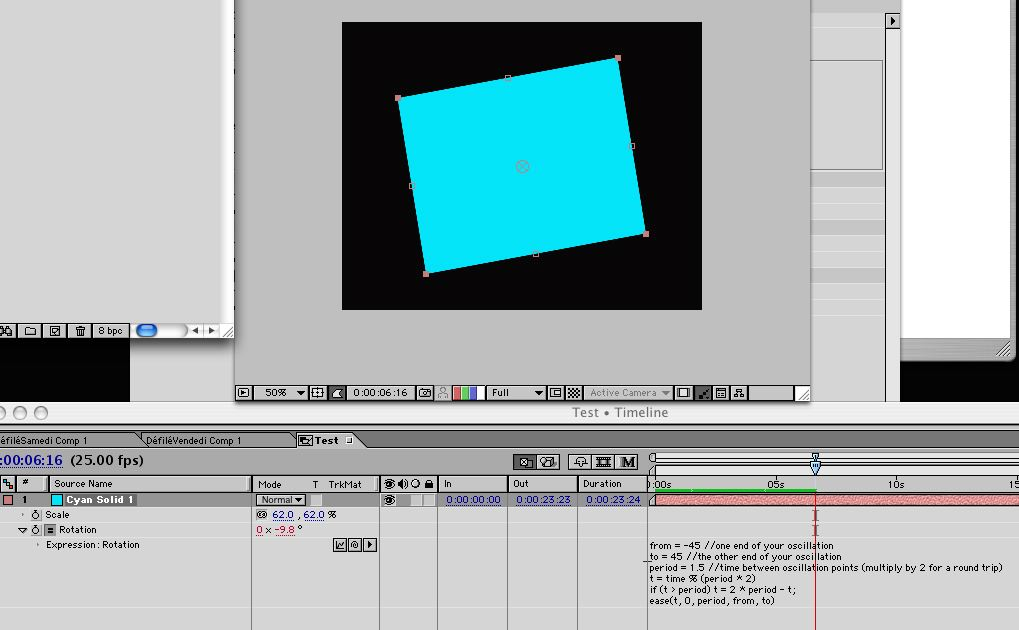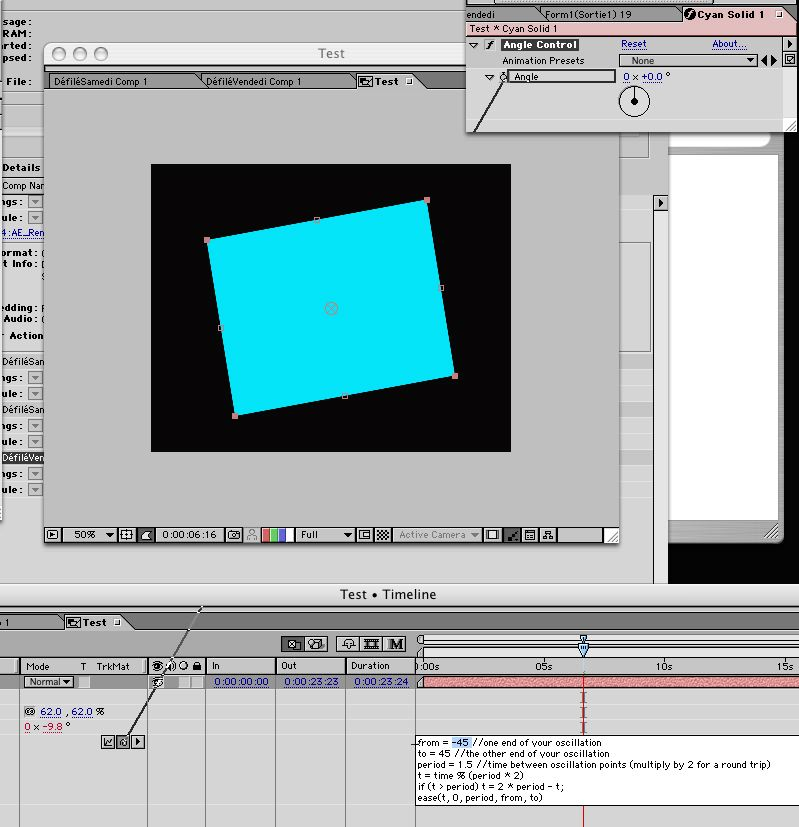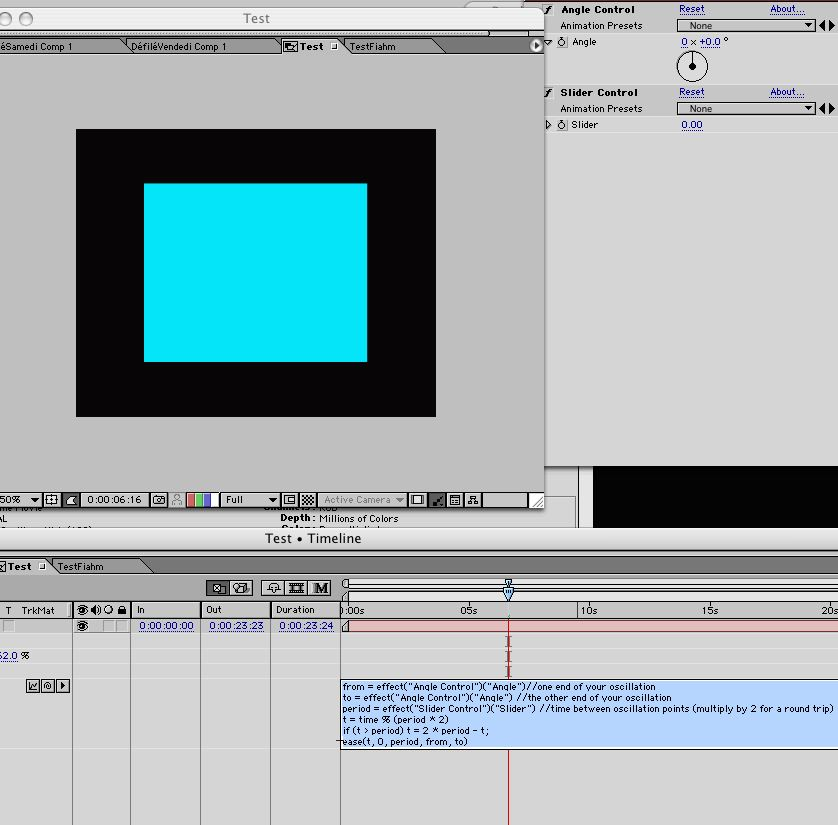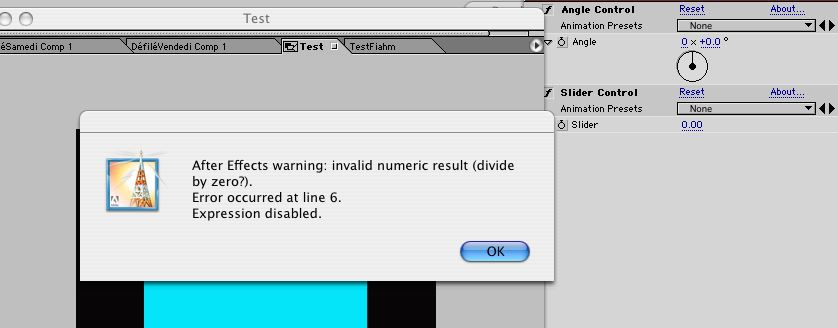Oscillator

Moderators: Disciple, zlovatt

Disciple
Posts: 137
Joined: June 5th, 2004, 8:05 am
Location: Los Angeles, CA
Contact:
This expression, to be applied to the rotation stream of a layer, will create a realistic oscillating look.
It was created by Brian Maffit and Michael Natkin. It can be found on the bonus CD of the Total Training AE 6 DVD.

from = -45; //one end of your oscillation
to = 45; //the other end of your oscillation
period = 1.5; //time between oscillation points (multiply by 2 for a round trip)
t = time % (period * 2)
if (t > period) t = 2 * period - t;
ease(t, 0, period, from, to)

What this expression does exactly is create an oscillating motion between two specified values (-45 and 45 in this case) over a specified period of time.

You can use Expression controls to animate these values. For the "from" and "to" variables you would use Angle Control or Slider Control, and for "period" you would use Slider Control.

However there is a catch to this : if you simply attach the Controls as they are, at their default values, you will get an error. But, there is a fix...

Here is a breakdown of how this works.

1/Type in the expression :2/Apply the expression controls (just select the value you want the control to replace by highlighting it in the expression field and pickwhip it to the control, that you have, of course, previously applied to the layer)3/This is what your expression should look like4/But hit return and you get this error :Now why is that? Well, the controls default to 0, so you are effectively dividing you values by 0. Funny thing is that the expression error is declared at line 6, whereas you can already see the error at line 4, where the first multiplication occurs.

So, what is workaround if you want to use expression controls here?
One way is to add a line to the expression, right below the "period" variable declaration :

if (period == 0) period = .01

Another workaround is to change you expression controls to another value than zero, and save the whole thing as an animation preset, which you can later apply without anymore errors popping up

--------------------------------

NOTE : you may have noticed a strange, unknown and unexpected sign in this expression, the % sign.
It occus in the following line :

t = time % (period * 2)

What this means is "mod". It means that if you divide the timeline into "chunks"

(period * 2)

Which in this case will be 3 seconds, time % will be a number that is the amount of seconds past the beginning of the current chunk.

Mod is something useful to use when doing periodic operations. A good way to see it in action is to apply this expression to a text layer in the source text stream :

time % (1.5 * 2)

What this will display is the time passing by, but only in periods of 3 seconds. Each time the timeline passes 3 seconds it will start at 0 again. So basically this is a sort of "measure" of periodic time.
afaj
Posts: 5
Joined: February 2nd, 2007, 9:30 pm
Very cool effect!

Two things that I'm noticing though (AF ver 7.0) are that I did not get the error message regarding the period, and also when I change the period slider control I don't see any difference in the effect.

Know why?